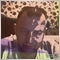• 概述
• 评论 (1)
• 评论 (1)
• 新特性

# CCI Global

### Description

A universal indicator CCI together with moving averages based on it. Depending on the need, moving averages can be disabled.

You can color the indicator lines and levels: lines of CCI, moving average lines and thresholds of overbought and oversold levels.

The indicator can be calculated as the standard one on the basis of a price or as a derivative on the basis of the following eighteen other indicators included in the standard package of the MetaTrader 4 terminal:

1. AD - an indicator of accumulation and distribution of volume.
2. OBV - On Balance Volume.
3. Volume - tick volume indicator.
4. AC - indicator of acceleration.
5. AO - Williams' oscillator.
6. BullsPower.
7. BearsPower.
8. BullsPower-BearsPower - the difference of the bulls power and bears power.
9. Force - Force Index indicator.
10. MFI - Money Flow Index.
11. ATR - Average True Range.
12. Momentum.
13. Moving Average.
14. RSI - Relative Strength Index.
15. ADX - Average Directional Movement indicator.
16. DeMarker.
17. Standard Deviation.
18. OsMA - moving average of oscillator.
19. MACD - moving average convergence/divergence.

Price is used by default.

### Purpose

The indicator can be used for manual or automated trading in an Expert Advisor. Values of indicator buffers of the double type can be used for automated trading:

1. The CCI line - buffer 0.
2. Fast MA line - buffer 1.
3. Slow MA line - buffer 2.

### Inputs

1. Calc_Method - the index of the indicator, based on which the CCI will be calculated (from 0 to 18). By default (more than 18) - a standard one based on the Close price.
2. CCI_Period - the period of the CCI indicator.
3. Periods - the period for calculating the basic indicator, based on which CCI will be calculated.
4. FastPeriod - the fast MA period of the basic indicator (for example MACD), based on which the CCI will be calculated.
5. SlowPeriod - the slow MA period of the basic indicator.
6. SignalPeriod - the signal line period of the basic indicator.
7. MA_Method - averaging method for the MA of the basic indicator and for MAs:
1. 7.1. MODE_SMA - 0.
2. 7.2. MODE_EMA - 1.
3. 7.3. MODE_SMMA - 2.
4. 7.4. MODE_LWMA - 3.
8. 8. Applied_Price - the used price of the basic indicator:
1. 8.1. PRICE_CLOSE - 0.
2. 8.2. PRICE_OPEN - 1.
3. 8.3. PRICE_HIGH - 2.
4. 8.4. PRICE_LOW - 3.
5. 8.5. PRICE_MEDIAN - 4.
6. 8.6. PRICE_TYPICAL - 5.
7. 8.7. PRICE_WEIGHTED - 6.
9. Mode - the index of the line (MAIN - 0 or SIGNAL - 1) of the basic indicator, based on which the CCI will be calculated.
10. Shift - the sift o the basic indicator relative to the price chart.
11. FastMA_Draw - the criterion of using in calculations and drawing the fast MA.
12. SlowMA_Draw - the criterion of using in calculations and drawing the slow MA.1913
2014.08.24 13:30

Реально отличный индикатор. Синергия в чистом виде.

Автору большое спасибо.

User's interface has been improved.